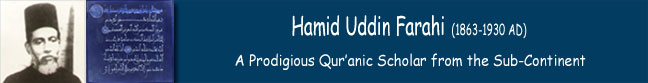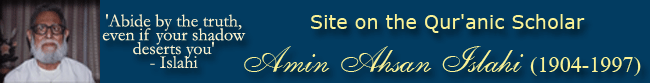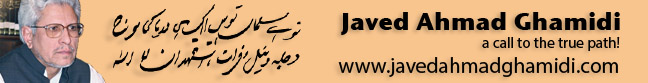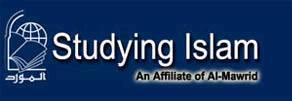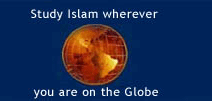Format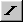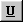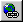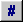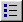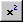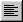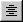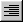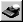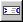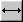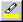Andale Mono Arial Arial Black Book Antiqua Century Gothic Comic Sans MS Courier New Georgia Impact Tahoma Times New Roman Trebuchet MS Script MT Bold Stencil Verdana Lucida Console 1 2 3 4 5 6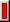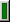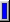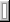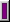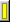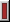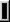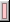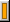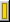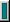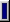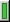Message Icon

Message

- Forum Code is ON
- HTML is OFF

 Smilies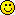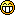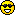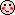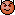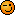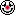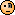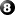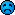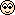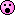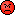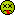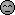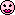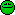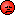Write in Urdu
اردو میں لکھيۓ
(For Urdu Forums Only)
Write here in Roman Urdu Preview

 A = آ a = ا
 B = N/A b = ب
 C = ث c = چ
 D = ڈ d = د
 E = N/A e = ع
 F = N/A f = ف
 G = غ g = گ
 H = ھ h = ح
 I = N/A i = ي
 J = ض j = ج
 K = خ k = ك
 L = N/A l = ل
 M = N/A m = م
 N = ں n = ن
 O = N/A o = ا
 P = N/A p = پ
 Q = N/A q = ق
 R = ڑ r = ر
 S = ص s = س
 T = ٹ t = ت
 U = ہ u = ء
 V = ظ v = ط
 W = N/A w = و
 X = ژ x = ش
 Y = N/A y = ے
 Z = ذ z = ز

Mode
Show Preview